﻿ Summary: Solving Triangles (All Types)

# Summary: Solving Triangles (All Types)

LESSON READ-THROUGH (Part 1 of 2)
by Dr. Carol JVF Burns (website creator)
Follow along with the highlighted text while you listen!
• PRACTICE (online exercises and printable worksheets)

To solve a triangle means to find all the angles and all the side lengths.
This section summarizes information related to solving triangles,
and presents ‘safe’ solution approaches for all triangle types.

Complete discussions are offered in the sections listed below; consult them as needed.

## Notation

Capital letters are used to denote angles/vertices.
Corresponding lowercase letters are used to denote lengths of opposite sides.
Thus:
• angle $\,D\,$ is opposite side $\,d\,$
• angle $\,E\,$ is opposite side $\,e\,$
• angle $\,F\,$ is opposite side $\,f\,$## Easy-to-use Triangle Solver

If you just need to solve a triangle reliably, here's an easy-to-use triangle solver.
It can also be used to check your work.

## Basic Tools/Ideas Useful for Solving Triangles

In any triangle:
• the angles sum to $\,180^\circ\,$
• angles are strictly between $\,0^\circ\,$ and $\,180^\circ\,$
• there is at most one angle that is $\,\ge 90^\circ\,$
• If a triangle has longest/medium/shortest sides, then:
• the longest side is opposite the biggest angle
• the medium side is opposite the medium angle
• the shortest side is opposite the smallest angle
• sides opposite equal angles are equal;
angles opposite equal sides are equal
Law of Sines
• Law of Sines:
In any triangle, the ratio of the sine of an angle to the length of its opposite side is constant: $$\cssId{s32}{\frac{\sin D}{d} = \frac{\sin E}{e} = \frac{\sin F}{f}}$$
• To use the Law of Sines, you must know an angle and its opposite side.
• If an unknown angle is ‘inside’ a sine, then you will ‘undo’ the sine with the arcsine function (abbreviated ‘$\,\arcsin\,$’).
• By definition, for $\,-1 \le x\le 1\,$:
$\,\arcsin(x) := \text{the angle between$\,-90^\circ\,$and$\,90^\circ\,$whose sine is$\,x\,$}\,.$
• In the context of solving triangles, $\,\arcsin(x)\,$ is always an angle in the interval $\,(0^\circ,90^\circ]\,.$
• If a calculator is in degree mode, then $\,\arcsin(x)\,$ is reported in degrees.
If a calculator is in radian mode, then $\,\arcsin(x)\,$ is reported in radians.
• If $\,D \le 90^\circ\,,$ then $\,D = \arcsin(\sin D)\,.$
If $\,D\,$ is obtuse, then $\,D = 180^\circ - \arcsin(\sin D)\,.$
• Caution:
If $\,D\,$ is an unknown angle in a triangle and you know $\,\sin D\,,$
then $\,\arcsin(\sin D)\,$ may not give you the angle you want!
• GOOD HABIT:
To avoid problems associated with the Law of Sines and the arcsine,
always use the Law of Sines with the shortest side available.
Memory device:   Law of Sines/Shorter
Law of Cosines
• Law of Cosines:
In any triangle, the square of a side is the sum of the squares of the other two sides,
minus twice the product of the other two sides times the cosine of the included angle:
$$\cssId{s53}{d^2 = e^2 + f^2 - 2ef\cos D}$$ (There are two other versions.)
• To use the Law of Cosines, you must know at least two sides.
Know two sides and the included angle? Solve for the remaining side.
Know three sides? Solve for an angle. (Good habit: solve for the biggest angle.)
• If an unknown angle is ‘inside’ a cosine, then you will ‘undo’ the cosine with the arccosine function (abbreviated ‘$\,\arccos\,$’).
• By definition, for $\,-1 \le x\le 1\,$:
$\,\arccos(x) := \text{the angle between$\,0^\circ\,$and$\,180^\circ\,$whose cosine is$\,x\,$}\,.$
• If a calculator is in degree mode, then $\,\arccos(x)\,$ is reported in degrees.
If a calculator is in radian mode, then $\,\arccos(x)\,$ is reported in radians.
• For all angles in triangles, $\,D = \arccos(\cos D)\,.$
• If $\,D\,$ is an unknown angle in a triangle and you know $\,\cos D\,,$
then $\,\arccos(\cos D)\,$ is always the angle you want!
• The Law of Cosines is ‘safer’ to use than the Law of Sines when solving triangles.
• GOOD HABIT:
Use the Law of Cosines with the biggest angle available (i.e., the angle opposite a longest side).
If a triangle has an obtuse angle, then the Law of Cosines will find it correctly, leaving only acute angles to be used with the Law of Sines.
This can help prevent ‘impossible’ triangles caused by an ‘uninformed’ use of the Law of Sines.
See ‘What Can Go Wrong?’ below.
Special Tools for Right Triangles
• the Pythagorean Theorem:
In a right triangle, the square of the hypotenuse is the sum of the squares of the other two sides: $$\cssId{s75}{d^2 = e^2 + f^2}$$ Here, $\,d\,$ is the hypotenuse (the side opposite the right angle).
It works the other direction, too: if a triangle satisfies $\,d^2 = e^2 + f^2\,,$ then it's a right triangle.
• Right Triangle Definitions (SOHCAHTOA):
$$\cssId{s80}{\sin\theta = \frac{\text{OPP}}{\text{HYP}}}\, \qquad \cssId{s81}{\cos\theta = \frac{\text{ADJ}}{\text{HYP}}}\, \qquad \cssId{s82}{\tan\theta = \frac{\text{OPP}}{\text{ADJ}}}$$
• There's a function that ‘undoes’ the tangent, similar to how the $\,\text{arcsin}\,$ ‘undoes’ the sine, and the $\,\text{arccos}\,$ ‘undoes’ the cosine.

By definition, for all real numbers $\,x\,$:
$\,\arctan(x) := \text{the angle strictly between$\,-90^\circ\,$and$\,90^\circ\,$whose tangent is$\,x\,$}\,.$

In the context of solving triangles, $\,\arctan(x)\,$ is always an angle in the interval $\,(0^\circ,90^\circ)\,.$
Arctan is ‘safe’ to use if you know the angle you're working with is acute.
Computational Considerations

 GOOD HABIT:   When solving triangles, select tools that use exact values, whenever possible. For example, suppose you're solving the right triangle shown at right. Green numbers are given (exact) values ($\,\color{green}{2}\,$ and $\,\color{green}{39^\circ}\,$). Black numbers are computed exact values ($\,51^\circ\,$). Red numbers are computed approximate values ($\,\color{red}{2.574}\,$). To compute $\,b\,,$ you should use $$\cssId{s97}{\tan 39^\circ = \frac{b}{2}}\qquad \cssId{s98}{\text{ or }} \qquad \cssId{s99}{\tan 51^\circ = \frac{2}{b}}$$ since they use only exact values. You should not use $$\cssId{s102}{\cos 51^\circ = \frac{b}{\color{red}{2.574}}}\qquad \cssId{s103}{\text{ or }}\qquad \cssId{s104}{\sin 39^\circ = \frac{b}{\color{red}{2.574}}}\qquad \cssId{s105}{\text{ or }}\qquad \cssId{s106}{b^2 + 2^2 = (\color{red}{2.574})^2}$$ since they use computed approximate values, which introduce more computational error. GOOD HABIT:   Use the full accuracy of your calculator, whenever possible. For example, if you've computed $\,2.574...\,$ and must use it later on, then use your calculator features (e.g., memory, recent values) to use all the calculator digits. Don't just type in the four digits $\,2.574\,$!Special Triangles

Be on the lookout for these special triangles.
When you recognize them, you should be able to fill in values from memory (no computations).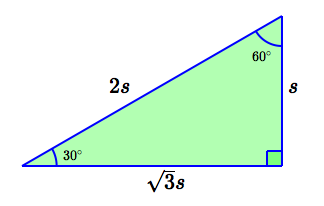$\,30^\circ\,$-$\,60^\circ\,$-$\,90^\circ\,$ triangle
 shortest side: opposite $\,30^\circ\,$ angle hypotenuse: twice the shortest side medium side: $\,\sqrt{3}\,$ times the shortest side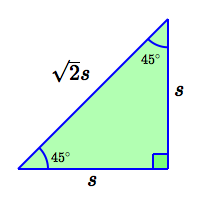$\,45^\circ\,$-$\,45^\circ\,$-$\,90^\circ\,$ triangle

hypotenuse is
$\,\sqrt{2}\,$ times the length of the leg

## Solving Triangles: What Can Go Wrong?

The following example illustrates what can go wrong when solving triangles, if good habits are not followed.

Consider a triangle with sides of length $\,3\,,$ $\,4\,$ and $\,6\,.$
Solve the triangle.

Faulty Solution:
• Let $\,D,\, E,\, F\,$ be the angles across from the sides of length $\,3,\, 4,\, 6\,.$
• Note that $\,6 < 3 + 4\,.$ By SSS, the triangle is unique.
• By the Law of Cosines: $$\begin{gather} \cssId{sb8}{ 3^2 = 4^2 + 6^2 - 2\cdot 4\cdot 6\cdot\cos D}\cr\cr \cssId{sb9}{\cos D \approx 0.8958} \cr\cr \cssId{sb10}{ D = \arccos(\cos D) \approx 26.38^\circ} \end{gather}$$
• By the Law of Sines: $$\begin{gather} \cssId{sb12}{\frac{\sin 26.38^\circ}{3} = \frac{\sin F}{6}}\cr\cr \cssId{sb13}{\sin F = \frac{6\sin 26.38^\circ}{3} \approx 0.8886}\cr\cr \cssId{sb14}{F = \arcsin(0.8886) \approx 62.70^\circ} \end{gather}$$
• $\,E = 180^\circ - 26.38^\circ - 62.70^\circ = 90.92^\circ$
• Summary:  ANGLES OPPOSITE SIDES $D = 26.38^\circ$ $d = 3$ $E = 90.92^\circ$ $e = 4$ $F = 62.70^\circ$ $f = 6$
This is an ‘impossible triangle’!
• The longest side is not opposite the biggest angle.
• The Law of Sines ratios are not all equal: $$\cssId{sb28}{\frac{\sin 26.38^\circ}{3} \approx 0.1481} \qquad \cssId{sb29}{\frac{\sin 90.92^\circ}{4} \approx 0.2500}$$
An application of just one of the two recommended ‘good habits’ would have prevented the impossible triangle. Here, both good habits are applied:

Correct Solution:
• Let $\,D,\, E,\, F\,$ be the angles across from the sides of length $\,3,\, 4,\, 6\,.$
• Note that $\,6 < 3 + 4\,.$ By SSS, the triangle is unique.
• Use the Law of Cosines with the longest side: $$\begin{gather} \cssId{sb36}{6^2 = 3^2 + 4^2 - 2\cdot 3\cdot 4\cdot\cos F}\cr\cr \cssId{sb37}{\cos F \approx -0.4583} \cr\cr \cssId{sb38}{F = \arccos(\cos F) \approx 117.3^\circ} \end{gather}$$
• Use the Law of Sines with the shortest side: $$\begin{gather} \cssId{sb40}{\frac{\sin 117.3^\circ}{6} = \frac{\sin D}{3}}\cr\cr \cssId{sb41}{\sin D = \frac{3\sin 117.3^\circ}{6} \approx 0.4443}\cr\cr \cssId{sb42}{D = \arcsin(0.4443) \approx 26.38^\circ} \end{gather}$$
• $\,E = 180^\circ - 117.3^\circ - 26.38^\circ = 36.32^\circ$
• Summary:  ANGLES OPPOSITE SIDES $D = 26.38^\circ$ $d = 3$ $E = 36.32^\circ$ $e = 4$ $F = 117.3^\circ$ $f = 6$
This is the correct triangle!

Note: At left, the corrections would be: $$\begin{gather} \cssId{sb56}{F = 180^\circ - \arcsin(0.8886) \approx 117.3^\circ}\cr\cr \cssId{sb57}{E = 180^\circ - 26.38^\circ - 117.3^\circ = 36.32^\circ} \end{gather}$$

## Congruence Theorems

 CONGRUENCE THEOREMS A unique triangle is determined by each of these configurations: SAS side-angle-side (two sides and an included angle) SSS side-side-side (three sides) AAS/SAA angle-angle-side (or, side-angle-angle) (two angles and a non-included side) ASA angle-side-angle (two angles and an included side)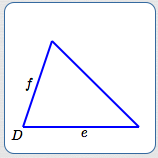If $$\begin{gather} \cssId{sb65}{e > 0}\cr \cssId{sb66}{f > 0}\cr \cssId{sb67}{0^\circ < D < 180^\circ} \end{gather}$$ then there exists a unique triangle having the two sides and included angle.Name the sides so that: $$\cssId{sb73}{0 < f \le e \le d}$$ Then, if $$\cssId{sb75}{d < e + f}$$ the triangle exists and is unique. That is: the longest side (if there is one) must be strictly less than the sum of the two other sides.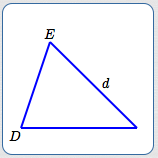If $$\begin{gather} \cssId{sb84}{0^\circ < D < 180^\circ}\cr \cssId{sb85}{0^\circ < E < 180^\circ}\cr \cssId{sb86}{D + E < 180^\circ}\cr \cssId{sb87}{d > 0} \end{gather}$$ then there exists a unique triangle having the two angles and non-included side. Note: Two angles uniquely determine the third. Three angles uniquely define the shape. One side uniquely defines the size.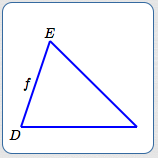If $$\begin{gather} \cssId{sb97}{0^\circ < D < 180^\circ}\cr \cssId{sb98}{0^\circ < E < 180^\circ}\cr \cssId{sb99}{D + E < 180^\circ}\cr \cssId{sb100}{f > 0} \end{gather}$$ then there exists a unique triangle having the two angles and included side. Note: Two angles uniquely determine the third. Three angles uniquely define the shape. One side uniquely defines the size.

## ‘SAFE’ Approaches for Solving Triangles

 ‘SAFE’ TRIANGLE-SOLVING STRATEGIES By using the guidelines here, you will avoid a mistake leading to an ‘impossible’ triangle. When it is safe to do so, the Law of Sines is preferred over the Law of Cosines, since it requires fewer computations. Once two angles are known, find the third angle using the fact that the angles sum to $\,180^\circ\,.$ SASUse the Law of Cosines to find $\,d\,.$ Use the Law of Sines with the shorter of $\,e\,$ or $\,f\,.$ Why? A triangle can have at most one obtuse angle. Therefore, at most one of $\,E\,$ or $\,F\,$ is obtuse. Whichever is opposite the shorter side is guaranteed to be acute. For acute angles: $$\cssId{sb119}{D = \arcsin(\sin D)}$$ SSSLet $\,d\,$ denote the longest side (if there is one). Use the Law of Cosines to find $\,D\,.$ If there is an obtuse angle, the Law of Cosines will find it correctly, and the remaining two angles will be acute. Use the Law of Sines to find a second angle. AAS/SAAUse the angle sum property to find the third angle. Use the Law of Sines twice to find the remaining two sides. Note: There is no danger in using the Law of Sines when the unknown is a side. ASAUse the angle sum property to find the third angle. Use the Law of Sines twice to find the remaining two sides. Note: There is no danger in using the Law of Sines when the unknown is a side.

OTHER TRIANGLE CONFIGURATIONS
AAA
• Infinitely many triangles have the same three angles. Therefore, you cannot ‘solve’ an AAA triangle.
• Triangles with the same angles are called similar triangles.
• Similar triangles have the same shape, but not necessarily the same size.
• Specifying the length of just one side determines the size.
This is the justification for the AAS and ASA congruence theorems.SSA/ASS
• The ‘SSA’ triangle condition (two sides and a non-included angle),
does not uniquely identify a triangle.
• Given two positive real numbers (two side lengths) and a degree measure strictly between $\,0^\circ\,$ and $\,180^\circ\,$ (the angle), there may be no triangle,
exactly one triangle, or two triangles that match the SSA information.
• The cut-off points for different behaviors are summarized below.
• You don't need to (and shouldn't) memorize the table below!
The mathematics will tell you which situation you're in (zero, one, or two triangles), providing you do the mathematics correctly!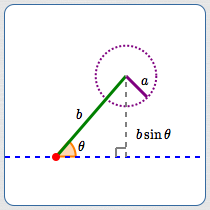Let: $$\begin{gather} \cssId{sb155}{a > 0}\cr\cr \cssId{sb156}{b > 0}\cr\cr \cssId{sb157}{0^\circ < \theta < 180^\circ} \end{gather}$$ Note: the side of length $\,a\,$ is opposite the known angle $\,\theta\,$
 $\theta < 90^\circ$ ($\,\theta\,$ is acute) $0 < a < b\sin\theta$ no triangle $a = b\sin\theta$ exactly one right triangle $b\sin\theta < a < b$ two different triangles $a = b$ exactly one isosceles triangle $a > b$ exactly one triangle $\theta \ge 90^\circ$ ($\,\theta\,$ is a right angle or obtuse) $0 < a \le b$ no triangle $a > b$ exactly one triangle

 SOLVING SSA CONFIGURATIONS: TWO APPROACHES Each step is illustrated with this example: Suppose a triangle has a $\,50^\circ\,$ angle opposite a side of length $\,6\,.$ A second side has length $\,7\,.$ Solve the triangle. Start by defining notation: Let $\,E\,$ be the angle opposite the side of length $\,7\,.$ Let $\,F,\,f\,$ be the remaining unknown angle and opposite side. USE THE LAW OF SINES:JUST BE CAREFUL USE THE LAW OF COSINES:SOLVE A QUADRATIC EQUATION Recognize the SSA configuration. Be aware of the potential for zero, one, or two triangles. Use the Law of Sines to get both potential angles: $$\begin{gather} \cssId{sb190}{\frac{\sin 50^\circ}{6} = \frac{\sin E}{7}}\cr\cr \cssId{sb191}{\sin E = \frac{7\sin 50^\circ}{6} \approx 0.8937} \cr\cr \cssId{sb192}{E_1 = \arcsin(0.8937) \approx 63.34^\circ}\cr \cssId{sb193}{E_2 = 180^\circ - 63.34^\circ = 116.66^\circ} \end{gather}$$ Get both potential third angles: $$\begin{gather} \cssId{sb195}{F_1 = 180^\circ - 50^\circ - 63.34^\circ = 66.66^\circ}\cr \cssId{sb196}{F_2 = 180^\circ - 50^\circ - 116.66^\circ = 13.34^\circ} \end{gather}$$ (Of course, discard a situation that results in a nonpositive angle.) Use the Law of Sines to find the remaining side(s): $$\begin{gather} \cssId{sb199}{\frac{\sin 50^\circ}{6} = \frac{\sin 66.66^\circ}{f_1}}\qquad \cssId{sb200}{\text{so}}\qquad \cssId{sb201}{f_1 \approx 7.19}\cr\cr \cssId{sb202}{\frac{\sin 50^\circ}{6} = \frac{\sin 13.34^\circ}{f_2}}\qquad \cssId{sb203}{\text{so}}\qquad \cssId{sb204}{f_2 \approx 1.81} \end{gather}$$ Compare the results. Different approaches may yield slightly different results. Greater accuracy at intermediate steps will help to minimize differences. Recognize the SSA configuration. Be aware of the potential for zero, one, or two triangles. Use the Law of Cosines starting with the side across from the known angle: $$\cssId{sb212}{6^2 = 7^2 + f^2 - 2\cdot 7\cdot f\cos 50^\circ}$$ Solve the quadratic equation. It may give zero, one, or two solutions for $\,f\,$: $$\begin{gather} \cssId{sb215}{f^2 - (14\cos 50^\circ)f + 13 = 0}\cr\cr \cssId{sb216}{f = \frac{14\cos 50^\circ \pm \sqrt{14^2\cos^2 50^\circ - 4(13)}}{2}}\cr\cr \cssId{sb217}{f_1 \approx 7.19}\cr \cssId{sb218}{f_2 \approx 1.81} \end{gather}$$ Use the Law of Sines on each configuration. Choose the shortest available side for each configuration. Note: first configuration: $\,e_1 = 7\,$ and $\,f_1 = 7.19\,,$ so $\,\color{red}{7} < 7.191\,$ second configuration: $\,e_2 = 7\,$ and $\,f_2 = 1.81\,,$ so $\,\color{green}{1.81} < 7\,$ $$\begin{gather} \cssId{sb226}{\frac{\sin 50^\circ}{6} = \frac{\sin E_1}{\color{red}{7}}}\qquad \cssId{sb227}{\text{so}}\qquad \cssId{sb228}{E_1 \approx 63.34^\circ}\cr\cr \cssId{sb229}{\frac{\sin 50^\circ}{6} = \frac{\sin F_2}{\color{green}{1.81}}}\qquad \cssId{sb230}{\text{so}}\qquad \cssId{sb231}{F_2 \approx 13.36^\circ} \end{gather}$$ Compute the remaining angles: $$\begin{gather} \cssId{sb233}{F_1 = 180^\circ - 50^\circ - 63.34^\circ = 66.66^\circ}\cr \cssId{sb234}{E_2 = 180^\circ - 50^\circ - 13.36^\circ = 116.64^\circ} \end{gather}$$
Master the ideas from this section